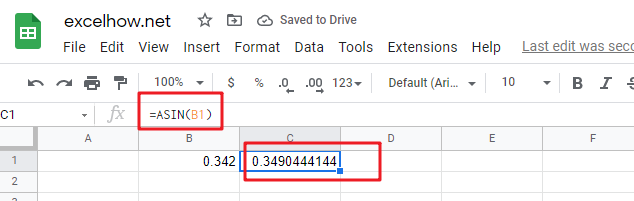This post will guide you how to use Google Sheets ASIN function with syntax and examples.

## Description

The Google Sheets ASIN function returns the arcsine value of a number.

The ASIN function can be used to get the inverse sine of a given number in google sheets. The purpose of this function is to return the inverse sine of a value in radians and its returned value is the angle in radians.

The ASIN function is a build-in function in Google Sheets and it is categorized as a MATH function.

## Syntax

The syntax of the ASIN function is as below:

= ASIN (number)

Where the ASIN function argument is:

• Number – This is a required argument.  A number between -1 and 1 that you want the arccosine value.

Note:

• ASIN is the inverse of SIN.
• If you wish to convert the result from radians to degrees, and you can multiply the result by 180/PI() or use the DEGREES function.

## Google Sheets ASIN Function Examples

The below examples will show you how to use google sheets ASIN function to return the arcsine value of a number.

#1 =ASIN(B1)